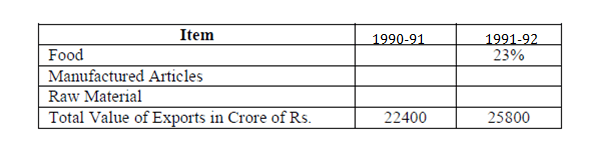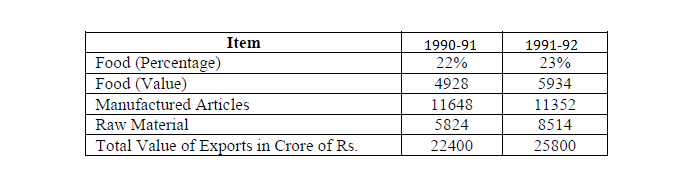DIRECTION : In 1990 – 91, value of exports of manufactured articles exceeds over the value of exports of raw materials by 100%. In 1991 – 92, the ratio of % of exports of raw material to that of exports of manufactured articles is 3 : 4. Value of Exports of food in 1991 – 92 exceeds the 1990– 91 figures by Rs. 1006 crore.Question 1.  During 1990 – 91, how much more raw material than food was exported?
(a) Rs. 2580 crore
(b) Rs. 896 crore
(c) Rs. 1986 crore
(d) Rs. 1852 crore

Question 2.  In 1990 –91 what percentage of total values of exports accounts for items related to food?
(a) 23%
(b) 29.2%
(c) 32%
(d) 22%

Question 3.  Value of exports of raw materials during 90 – 91 was how much percent less than that for 91 – 92?
(a) 39
(b) 46.18
(c) 7
(d) 31.6

Question 4. The change in value of exports of manufactured articles from 1990 – 91 to 1991 – 92 is
(a) 296 crore
(b) 629 crore
(c) 2064 crore
(d) 1792 crore

Answer for 1 to 4 From the data that is given we can find the following data- The starting point for filling the data in the vacant columns of the given table should from food’ export value. We know that in 1991-92, value of food export is 23% of 25800= 5934. Now as it is given that this figure is 1006 cr more than last year’s value, hence value of food in 1990-91 is 4928. (The rest of the explanation of how the following values were arrived at is given after the table).The Value of Manufactured articles & Raw materials exports In 90-91, = (22400 – 4928) = Rs.17472 crores.  Since Export in manufactured goods is twice that of raw materials,

Therefore the ratio of export of manufactured goods and raw materials is 2:1

Therefore export of manufactured goods = Rs.11648 crores and Raw materials = Rs.5824 crores.

Hence the difference between raw material and food = (5824 – 4928) = Rs.896 crores.

The exports related to food in 91-92 = 0.23 x 25800 = 5934.

Therefore the exports related to food 1990-91 = (5934 – 1006) = 4928.

Therefore the percentage food related exports in 90-91 = 4928/22400 = 22%.

The combined percentage of Manufactured articles and Raw materials in 91-92 = 77%

The ratio will be 4 : 3.

Therefore the percentage of Manufactured articles export = 44%

And the percentage of Raw materials export = 33%.

Therefore value of manufactured articles = 0.44 x 25800 = Rs.11352 crores

And the value of Raw materials = Rs.8514 crores.

Therefore the percentage difference between the value of Raw materials between 90-91 and 91-92 = [(8514 – 5824)/8514] × 100 = 31.6%